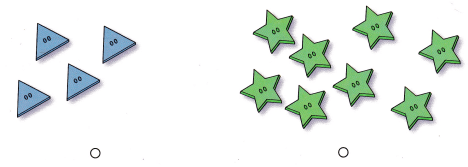# Texas Go Math Kindergarten Lesson 6.3 Answer Key Compare by Counting Sets to 10

Refer to our Texas Go Math Kindergarten Answer Key Pdf to score good marks in the exams. Test yourself by practicing the problems from Texas Go Math Kindergarten Lesson 6.3 Answer Key Compare by Counting Sets to 10.

## Texas Go Math Kindergarten Lesson 6.3 Answer Key Compare by Counting Sets to 10

Explore

DIRECTIONS: Look at the sets of objects. Count how many in each set. Use cubes to keep track of the objects that have been counted. Trace the numbers that show how many. Compare the numbers. Circle the greater number.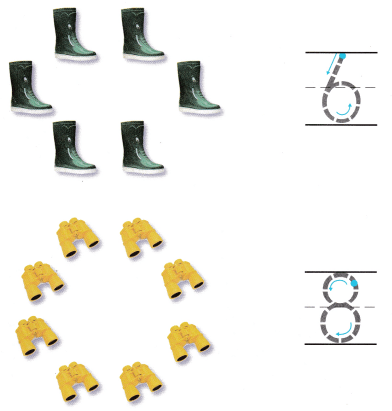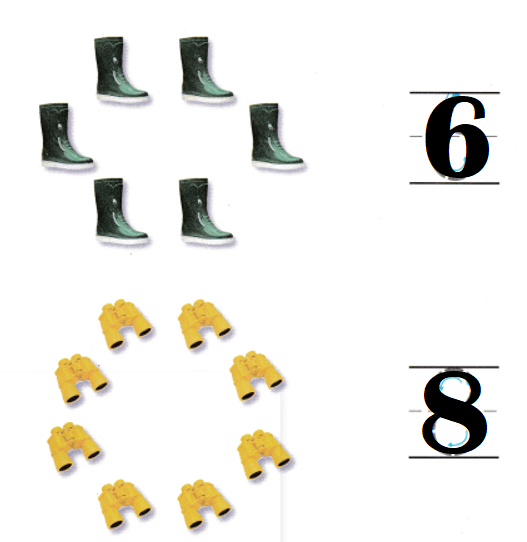Explanation:
Number of boots = 6.
Number of binoculars = 8.
6 < 8.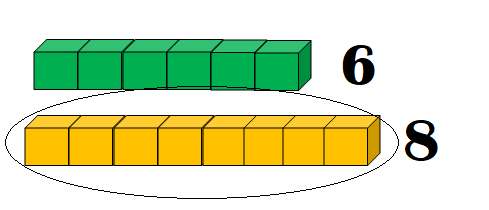Share and Show

DIRECTIONS: 1-3. Count how many ¡n each set. Write the number of objects in each set. Compare the numbers. Circle the greater number.

Question 1.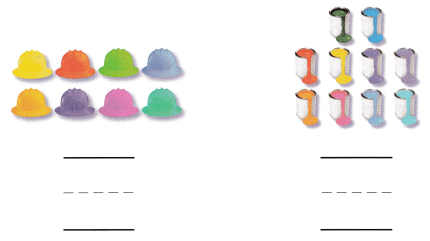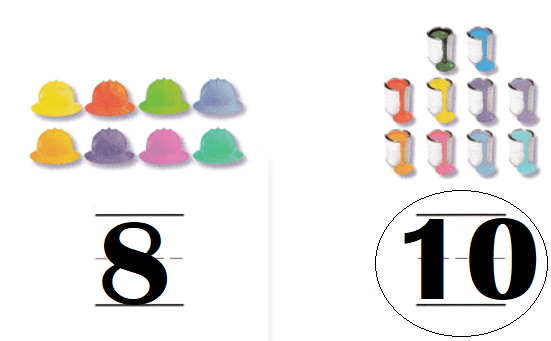Explanation:
Number of hats = 8.
Number of color bottles = 10.
8 < 10.

Question 2.

Explanation:

Question 3.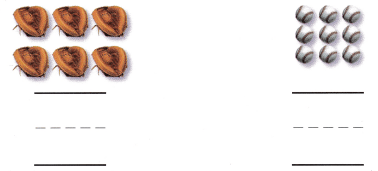Explanation:
Number of insects = 6.
Number of balls = 9.
6 < 9.

DIRECTIONS: 4.-6. Count how many ¡n each set. Write the number of objects in each set. Compare the numbers. Circle the number that is less.

Question 4.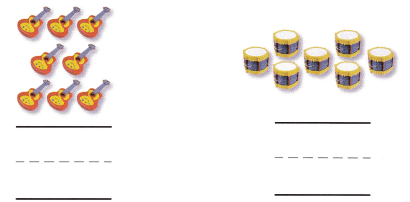Explanation:
Number of violin = 8.
Number of drums = 7.
8 > 7.

Question 5.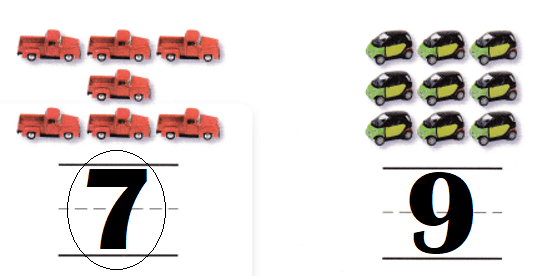Explanation:
Number of trucks = 7.
Number of cars = 9.
7 < 9.

Question 6.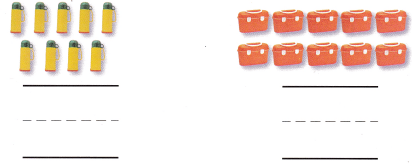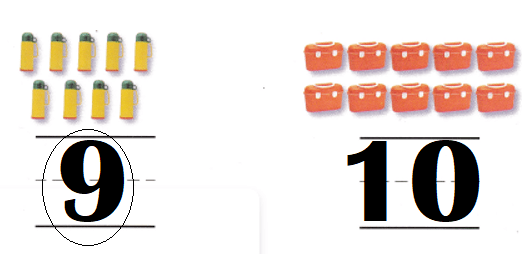Explanation:
Number of bottles = 9.
Number of purses = 10.
9 < 10.

HOME ACTIVITY • Show your child two sets of up to 10 objects. Have your child write the number of objects in each set. Ask which number is greater.
Number of dots are more than the number of cubes.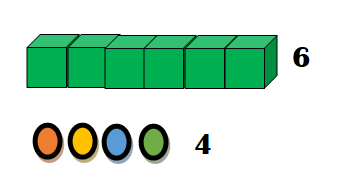Explanation:
My child bought cubes and dots.
Number of cubes = 6.
Number of dots = 4.
6 > 4.

DIRECTIONS: 7. Megan bought hats and gifts for the party. Count how many in each set. Write the number of objects in each set. Compare the numbers. Tell a friend about the sets. 8. Choose the correct answer. Compare the sets. Which set has more backpacks?

Problem Solving
Question 7.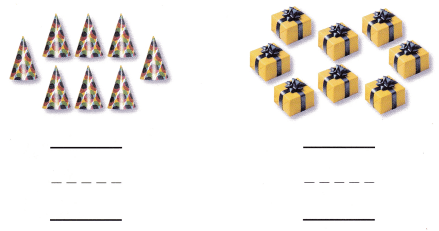Number of cone-shaped hats are equal to number of gifts.Explanation:
Number of cone-shaped hats = 8.
8 = 8.

Question 8.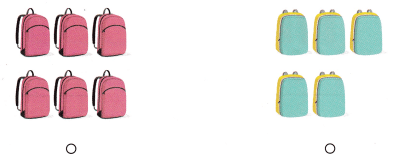Explanation:
Number of purple bags = 6.
Number of blue bags = 5.
6 > 5.

### Texas Go Math Kindergarten Lesson 6.3 Homework and Practice Answer Key

DIRECTIONS: 1-2. Count how many in each set. Write the number of objects in each set. Compare the numbers. Circle the greater number.

Question 1.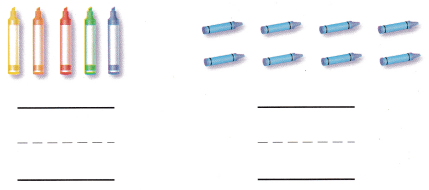Explanation:
Number of multiple colored crayons = 5.
Number of blue crayons = 8.
5 < 8.

Question 2.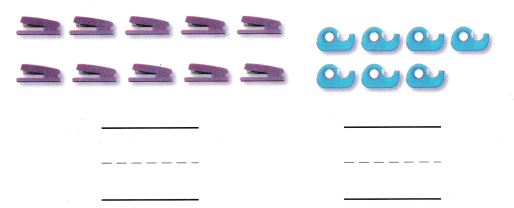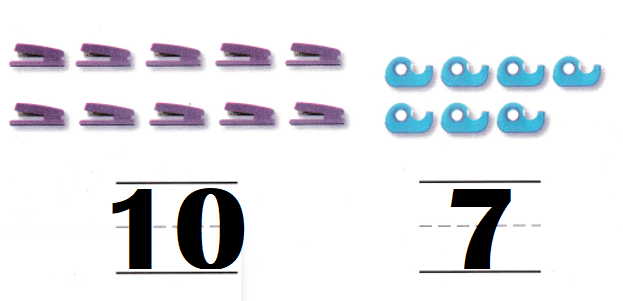Explanation:
Number of samplers = 10.
Number of tape machine = 7.
10 > 7.

DIRECTIONS: Choose the correct answer. 3-5. Compare the sets. Which set has fewer?

Lesson Check
Question 3.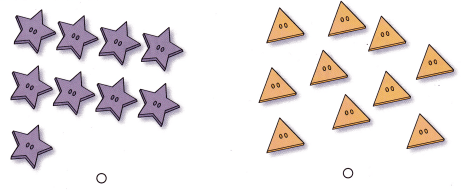Explanation:
Number of stars buttons = 9.
Number of triangle buttons = 10.
9 < 10.

Question 4.Explanation:
Number of square buttons = 7.
Number of circle buttons = 5.
7 > 5.

Question 5.